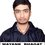# What Is Temperature ?

Look friends! Temperature totally depends on the Internal Energy. It is quite untrue that addition of heat will necessarily increase the temperature of the system under study. If heat energy is supplied it can be counter balanced by an equal amount of work being done by the system! It's just like you receive cash from one hand and then you spend an equal amount from your savings leading to no net profit.
The temperature of a body is a measure of it's total internal energy and nothing else ! The temperature of a system can be varied due to heat exchange or due to work being done on or being done by the system.
One example is that suppose we heat a container of gas (the container has a movable lid). Then the gas molecules expand against the external pressure and may end up doing an equivalent amount of work as the supplied heat energy. Hence in the end the gas did not gain any energy! So, the temperature did not change despite the fact that heat was supplied to the system!Note by Mayank Holmes
5 years, 8 months ago

This discussion board is a place to discuss our Daily Challenges and the math and science related to those challenges. Explanations are more than just a solution — they should explain the steps and thinking strategies that you used to obtain the solution. Comments should further the discussion of math and science.

When posting on Brilliant:

• Use the emojis to react to an explanation, whether you're congratulating a job well done , or just really confused .
• Ask specific questions about the challenge or the steps in somebody's explanation. Well-posed questions can add a lot to the discussion, but posting "I don't understand!" doesn't help anyone.
• Try to contribute something new to the discussion, whether it is an extension, generalization or other idea related to the challenge.

MarkdownAppears as
*italics* or _italics_ italics
**bold** or __bold__ bold
- bulleted- list
• bulleted
• list
1. numbered2. list
1. numbered
2. list
Note: you must add a full line of space before and after lists for them to show up correctly
paragraph 1paragraph 2

paragraph 1

paragraph 2

[example link](https://brilliant.org)example link
> This is a quote
This is a quote
    # I indented these lines
# 4 spaces, and now they show
# up as a code block.

print "hello world"
# I indented these lines
# 4 spaces, and now they show
# up as a code block.

print "hello world"
MathAppears as
Remember to wrap math in $$ ... $$ or $ ... $ to ensure proper formatting.
2 \times 3 $2 \times 3$
2^{34} $2^{34}$
a_{i-1} $a_{i-1}$
\frac{2}{3} $\frac{2}{3}$
\sqrt{2} $\sqrt{2}$
\sum_{i=1}^3 $\sum_{i=1}^3$
\sin \theta $\sin \theta$
\boxed{123} $\boxed{123}$